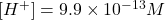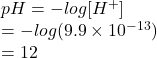## Given a solution with a hydrogen ion concentration of 9.9 x10-13 M, calculate the pОН. .

Question

Given a solution with a hydrogen ion concentration of 9.9 x10-13 M, calculate the
pОН. .

in progress 0
1 month 2021-08-15T05:28:17+00:00 1 Answers 3 views 0

1. Answer: The pOH value is 2.

Explanation:

Given:pH is defined as the negative logarithm of hydrogen ion concentration.

Hence, pH of the given solution is calculated as follows.The relation between pH and pOH is as follows.

pH + pOH = 14

Therefore, pOH is calculated as follows.

pH + pOH = 14

12 + pOH = 14

pOH = 14 – 12

= 2

Thus, we can conclude that the pOH value is 2.